# Deducible rule

(diff) ← Older revision | Latest revision (diff) | Newer revision → (diff)
A meta-mathematical theorem (cf. Meta-theorem) from which it is allowed by the use of a finite number of derivations (cf. Derivation, logical) from hypotheses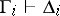(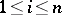,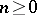) to show that a formulais derivable from the hypotheses; the derivationsare called auxiliary derivations of the deducible rule, while the conclusion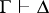is called the resulting derivation. A deducible rule is a special case of a sound rule. The most important examples of deducible rules are the deduction theorem, the rule of reductio ad absurdum, and other rules governing the introduction and elimination of logical symbols, such as the rules for the introduction of the disjunction: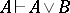and(for these deducible rules the number of auxiliary derivations is zero), and of elimination of disjunction: from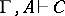and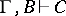follows that. In a number of cases, deducible rules have the following structure: A calculus is extended and strengthened, and the deducibility in the new calculus implies certain consequences regarding the deducibility in the basic calculus. Such deducible rules arise, in particular, in the elimination of descriptive definitions (definitions modelling the extensions of concepts and notations in constructing a mathematical theory). The available apparatus of deducible rules serves to bring the conversion methods with formal conclusions much nearer to specific mathematical reasoning.